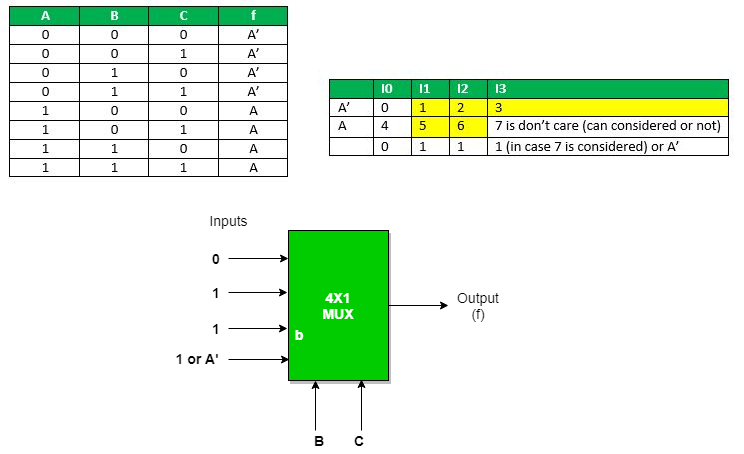# Multiplexers in Digital Logic

It is a combinational circuit which have many data inputs and single output depending on control or select inputs.​ For N input lines, log n (base2) selection lines, or we can say that for 2n input lines, n selection lines are required. Multiplexers are also known as “Data n selector, parallel to serial convertor, many to one circuit, universal logic circuit​”. Multiplexers are mainly used to increase amount of the data that can be sent over the network within certain amount of time and bandwidth.Now the implementation of 4:1 Multiplexer using truth table and gates.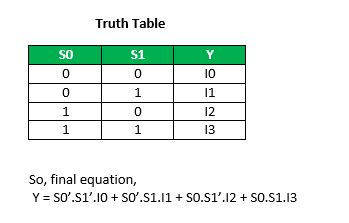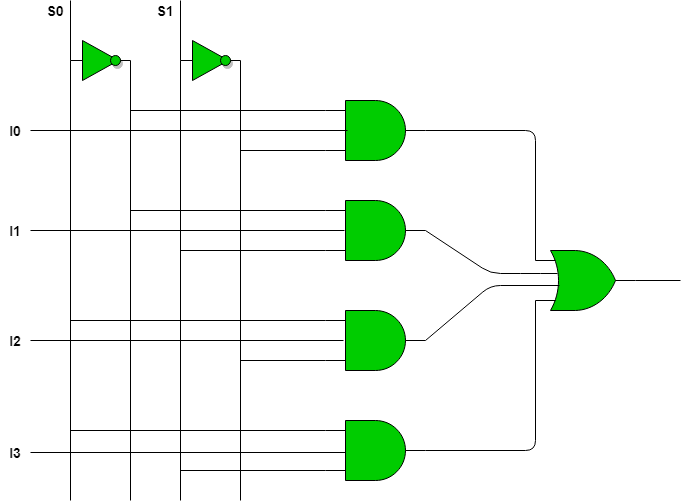Multiplexer can act as universal combinational circuit. All the standard logic gates can be implemented with multiplexers.

a) Implementation of NOT gate using 2 : 1 Mux

NOT Gate :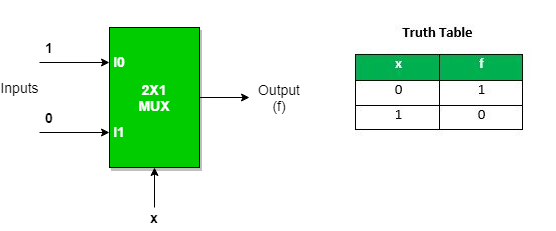We can analyze it
Y = x’.1 + x.0 = x’
It is NOT Gate using 2:1 MUX.
The implementation of NOT gate is done using “n” selection lines. It cannot be implemented using “n-1” selection lines. Only NOT gate cannot be implemented using “n-1” selection lines.

b) Implementation of AND gate using 2 : 1 Mux

AND GATE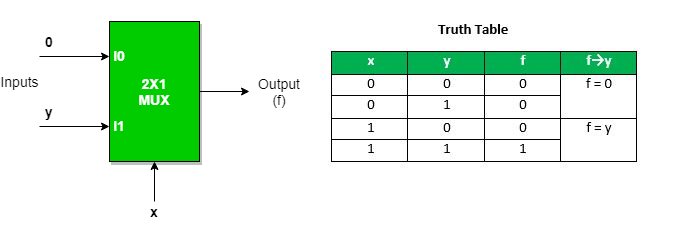This implementation is done using “n-1” selection lines.

c) Implementation of OR gate using 2 : 1 Mux using “n-1” selection lines.

OR GATE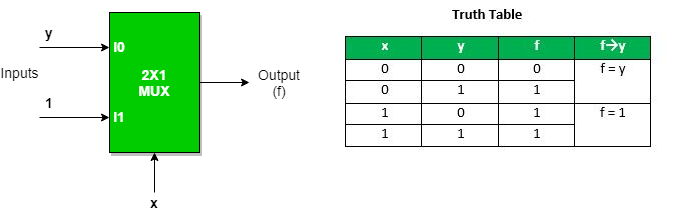Implementation of NAND, NOR, XOR and XNOR gates requires two 2:1 Mux. First multiplexer will act as NOT gate which will provide complemented input to the second multiplexer.

d) Implementation of NAND gate using 2 : 1 Mux

NAND GATE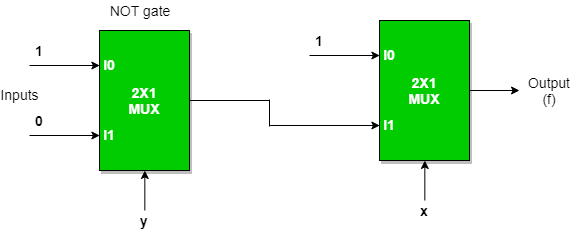e) Implementation of NOR gate using 2 : 1 Mux

NOR GATEf) Implementation of EX-OR gate using 2 : 1 Mux

EX-OR GATE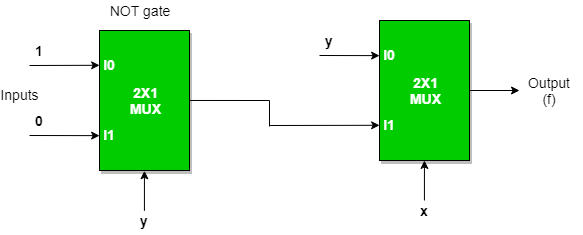g) Implementation of EX-NOR gate using 2 : 1 Mux

EX-NOR GATE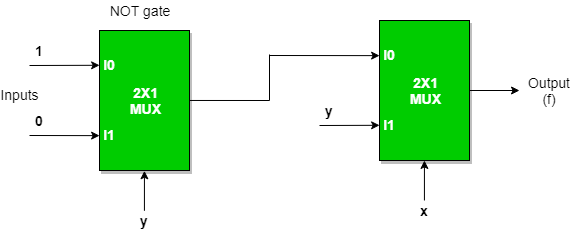Implementation of Higher order MUX using lower order MUX

a) 4 : 1 MUX using 2 : 1 MUX

Three(3) ​2 : 1 MUX are required to implement 4 : 1 MUX.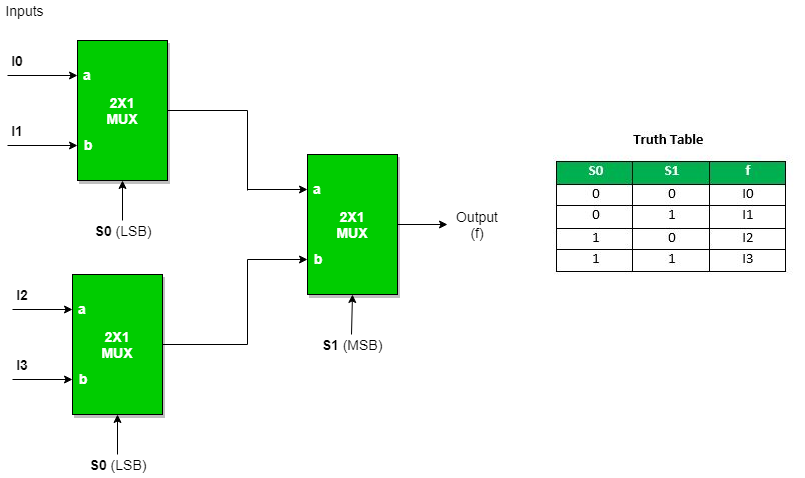Similarly,

While 8 : 1 MUX require seven(7) ​2 : 1 MUX, 16 : 1 MUX require fifteen(15) ​2 :1 MUX, 64 : 1 MUX requires sixty three(63)​ 2 : 1 MUX.
Hence, we can draw a conclusion,
2n : 1 MUX requires (2n- 1) 2 : 1 MUX.

b) 16 : 1 MUX using 4 : 1 MUX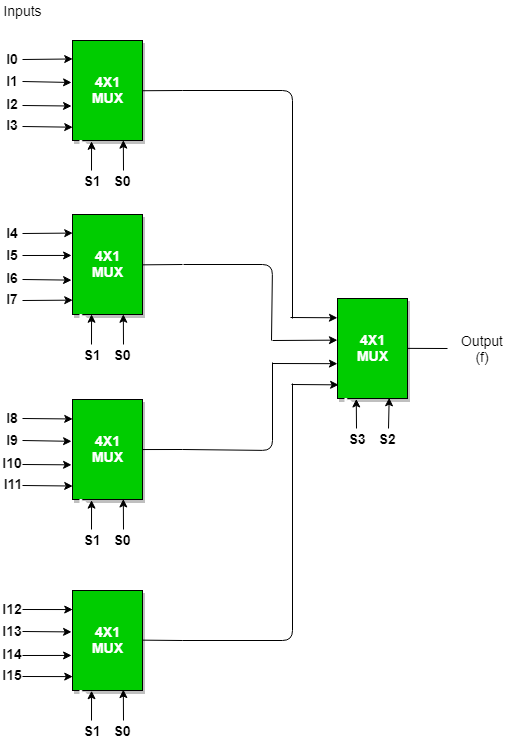In general, to implement B : 1 MUX using A : 1 MUX , one formula is used to implement the same.
B / A = K1,
K1/ A = K2,
K2/ A = K3

………………

KN-1 / A = KN = 1 (till we obtain 1 count of MUX).

And then add all the numbers of MUXes = K1 + K2 + K3 + …. + KN.
For example​ : To implement 64 : 1 MUX using 4 : 1 MUX
Using the above formula, we can obtain the same.
64 / 4 = 16
16 / 4 = 4
4 / 4 = 1 (till we obtain 1 count of MUX)
Hence, total number of 4 : 1 MUX are required to implement 64 : 1 MUX = 16 + 4 + 1 = 21.

An example to implement a boolean function if minimal and don’t care terms are given using MUX​.
f ( A, B, C) = Σ ( 1, 2, 3, 5, 6 ) with don’t care (7) using 4 : 1 MUX using as
a) AB as select : ​Expanding the minterms to its boolean form and will see its 0 or 1 value in Cth place so that they can be placed in that manner.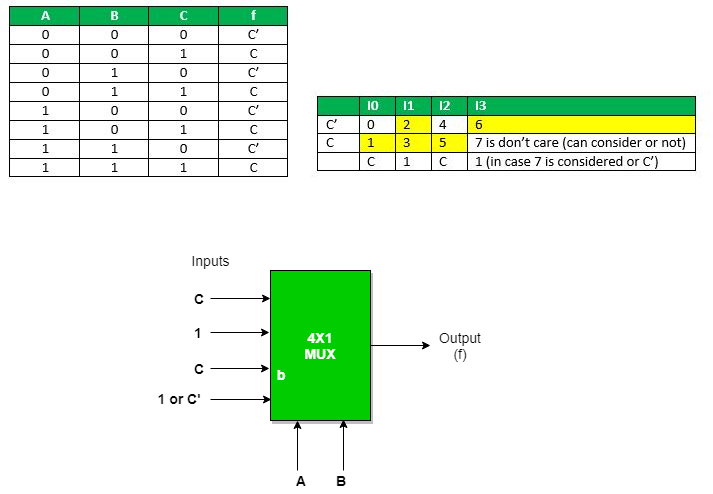b) AC as select : Expanding the minterms to its boolean form and will see its 0 or 1 value in Bth place so that they can be place in that manner.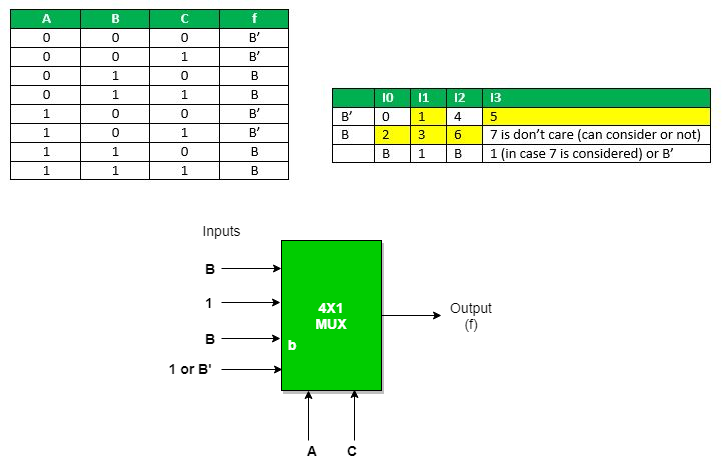c) BC as select : ​Expanding the minterms to its boolean form and will see its 0 or 1 value in Ath place so that they can be place in that manner.